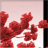# QlikView App Dev

Discussion Board for collaboration related to QlikView App Development.

Announcements
Join us at the Cloud Data and Analytics Tour! REGISTER TODAY
cancel
Showing results for
Search instead for
Did you mean:Specialist

## Incorrect dates are getting displayed

Hi ,

I have scenario where I have data as below,

 DATE TestNum Value 20150911 52 -0.09958 20150918 53 -0.01105 20150925 54 -0.14401 20151002 55 0.14401 20151009 56 0.05528 20151016 57 0.07742 20151023 58 0.09958 20151030 59 -0.11465

here, TestNum I have taken Autonumber based on date and sorted dates asc

here I am showing data for last 8 weeks date based on selected date.

Selected date is 20151030

from TestNum i have calculated current week as only(TestNum) and prior8week as only(TestNum)-7

now, I have calculated value also highest value and min value based on 8 week range but, now,

i have to display date corresponding to max value in textbox and same for minimum

in this, case my maxdate should be 20151002 and mindate should be 20150925.

Can some one help

1 Solution

Accepted SolutionsMVP

May be this

FirstSortedValue({<TestNum = {">=\$(=only(TestNum)-7)<=\$(=only(TestNum))"}>} DATE, -Aggr(Sum({<TestNum = {">=\$(=only(TestNum)-7)<=\$(=only(TestNum))"}>} Value), DATE))

FirstSortedValue({<TestNum = {">=\$(=only(TestNum)-7)<=\$(=only(TestNum))"}>} DATE, Aggr(Sum({<TestNum = {">=\$(=only(TestNum)-7)<=\$(=only(TestNum))"}>} Value), DATE))

4 RepliesMVP

May be this

FirstSortedValue({<TestNum = {">=\$(=only(TestNum)-7)<=\$(=only(TestNum))"}>} DATE, -Aggr(Sum({<TestNum = {">=\$(=only(TestNum)-7)<=\$(=only(TestNum))"}>} Value), DATE))

FirstSortedValue({<TestNum = {">=\$(=only(TestNum)-7)<=\$(=only(TestNum))"}>} DATE, Aggr(Sum({<TestNum = {">=\$(=only(TestNum)-7)<=\$(=only(TestNum))"}>} Value), DATE))Specialist
Author

It's showing me the same date which I have selectedSpecialist
Author

It's working when I excluded selected date field. Thank you!!Specialist
Author

Hi Sunny,

I am facing an issue with firstsortedvalue() below expression.Now, I am having two same values for different dates but, in this case I should get latest date.

So., I have used distinct in firstsortedvalue() but, it seems sometimes working and sometimes not.

Could you please suggest on the same.